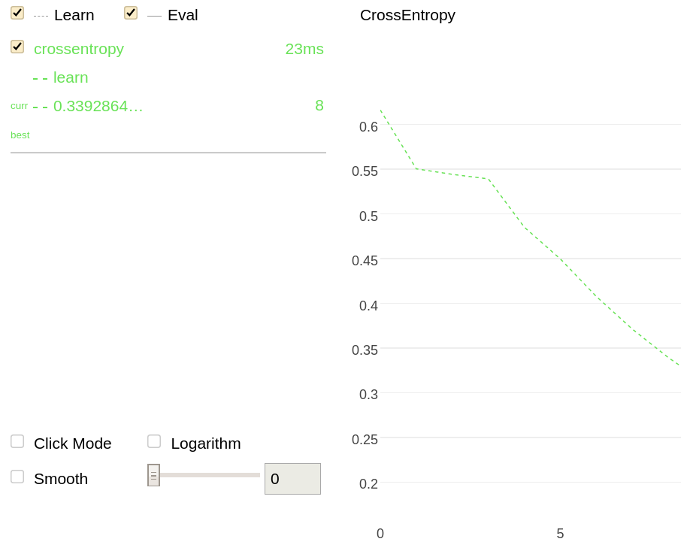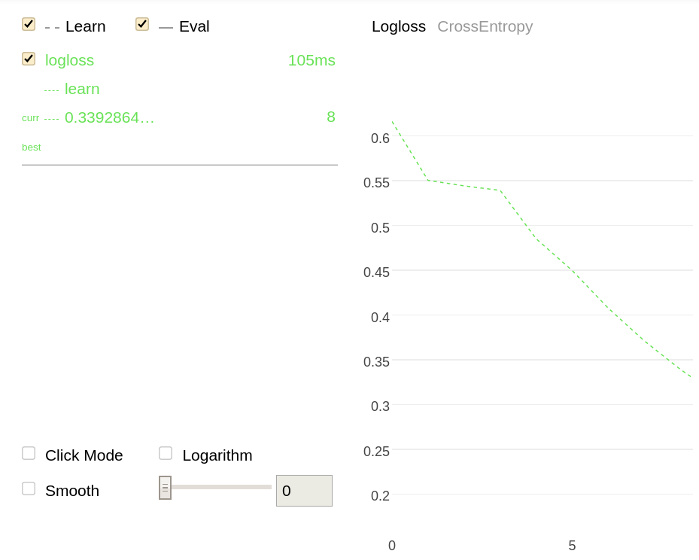# MetricVisualizer

``````class MetricVisualizer(train_dirs,
subdirs=False)``````

## Purpose

Plot information regarding training, metric evaluations or cross-validation runs on a single chart. One chart can contain information regarding one or several runs depending on the input information. The charts can be either plotted in real time while the trainings are performed, or after the trainings are over.

## Parameters

Parameter Possible types Description Default value
train_dirs
• string
• list of strings

The directory or the list of directories to read the files generated during training.

catboost_info
subdirs bool

Gather and read data from the specified directories and all subdirectories.

False (the data for charts is gathered from the specified directories only)
Parameter Possible types Description Default value
train_dirs
• string
• list of strings

The directory or the list of directories to read the files generated during training.

catboost_info
subdirs bool

Gather and read data from the specified directories and all subdirectories.

False (the data for charts is gathered from the specified directories only)

## Methods

Method Description
start

Plot metrics for all training, metric evaluations and cross-validation runs that have logs in the given directory.

Method Description
start

Plot metrics for all training, metric evaluations and cross-validation runs that have logs in the given directory.

## Gather data from the specified directory only

1. Train a model from the root of the file system (/):
``````from catboost import CatBoostClassifier

cat_features = [0,1,2]

train_data = [["a", "b", 1, 4, 5, 6],
["a", "b", 4, 5, 6, 7],
["c", "d", 30, 40, 50, 60]]

train_labels = [1,1,0]

model = CatBoostClassifier(iterations=20,
loss_function = "CrossEntropy",
train_dir = "crossentropy")

model.fit(train_data, train_labels, cat_features)
predictions = model.predict(train_data)``````
2. Plot a chart using the information regarding the previous training (from the crossentropy directory):
``````import catboost

w = catboost.MetricVisualizer('/crossentropy/')
w.start()``````

The following is a chart plotted with Jupyter Notebook for the given example.## Gather and read data from all subdirectories

1. Train two models from the root of the file system (/):
1. ``````from catboost import CatBoostClassifier

cat_features = [0,1,2]

train_data = [["a", "b", 1, 4, 5, 6],
["a", "b", 4, 5, 6, 7],
["c", "d", 30, 40, 50, 60]]

train_labels = [1,1,0]

model = CatBoostClassifier(iterations=20,
loss_function = "CrossEntropy",
train_dir = "crossentropy")

model.fit(train_data, train_labels, cat_features)
predictions = model.predict(train_data)``````
2. ``````from catboost import CatBoostClassifier

cat_features = [0,1,2]

train_data = [["a", "b", 1, 4, 5, 6],
["a", "b", 4, 5, 6, 7],
["c", "d", 30, 40, 50, 60]]

train_labels = [1,1,0]

model = CatBoostClassifier(iterations=20,
train_dir = "logloss")

model.fit(train_data, train_labels, cat_features)
predictions = model.predict(train_data)``````
2. Plot charts using the information from all subdirectories (crossentropy and logloss) of the root of the file system:
``````import catboost

w = catboost.MetricVisualizer('/', subdirs=True)
w.start()``````

The following is a chart plotted with Jupyter Notebook for the given example.